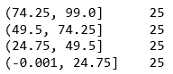# Sort and Segment Your Data into Bins to Get Sorted RangesWhen You Are Looking for a Range Not an Exact Value, a Grade Not a Score. Binning the data can be a very useful strategy while dealing with numeric data to understand certain trends.

Binning the data can be a very useful strategy while dealing with numeric data to understand certain trends. Sometimes, we may need an age range, not the exact age, a profit margin not profit, a grade not a score. The Binning of data is very helpful to address those. Pandas library has two useful functions cut and qcut for data binding. But sometimes they can be confusing. In this article, I will try to explain the use of both in detail.

## Binning

To understand the concept of binning, we may refer to a histogram. I am going to use a student performance dataset for this tutorial. Please feel free to download the dataset from this link:

Import the necessary packages and the dataset now.

``````import pandas as pd
import numpy as np
import seaborn as snsdf = pd.read_csv('StudentsPerformance.csv')``````

Using the dataset above, make a histogram of the math score data:

``df['math score'].plot(kind='hist')``

We did not mention any number of bins here but behind the scene, there was a binning operation. Math scores have been divided into 10 bins like 20–30, 30–40. There are many scenarios where we need to define the bins discretely and use them in the data analysis.

## qcut

This function tries to divide the data into equal-sized bins. The bins are defined using percentiles, based on the distribution and not on the actual numeric edges of the bins. So, you may expect the exact equal-sized bins in simple data like this one

``pd.Series(pd.qcut(range(100), 4)).value_counts()``In this example, we just gave a range from 0 to 99 and asked the qcut function to divide it into 4 equal bins. It made 4 equal bins of 25 elements each. But when the data is bigger and the distribution is a bit complex, the value_counts in each bin may not be equal as the bins are defined using the percentiles.

Here are some example use cases of qcut:

## Data Science With Python Training | Python Data Science Course | Intellipaat

🔵 Intellipaat Data Science with Python course: https://intellipaat.com/python-for-data-science-training/In this Data Science With Python Training video, you...

## Master Pandas’ Groupby for Efficient Data Summarizing And Analysis

Learn to group the data and summarize in several different ways, to use aggregate functions, data transformation, filter, map.

## Data Analysis | Data Analysis Projects | Data Science Projects | Exploratory Data Analysis | Pandas

In this tutorial, you will know about the TED TALKS DATA ANALYSIS project from scratch.

## Python for Data Science | Data Science With Python | Python Data Science Tutorial

🔥Intellipaat Python for Data Science Course: https://intellipaat.com/python-for-data-science-training/In this python for data science video you will learn e...

## An introduction to exploratory data analysis in python

Many a time, I have seen beginners in data science skip exploratory data analysis (EDA) and jump straight into building a hypothesis function or model. In my opinion, this should not be the case.